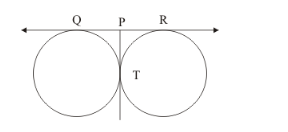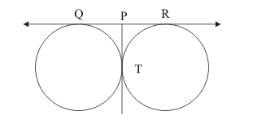# In the given figure, two equal circles touch each other at T, if OP = 4.5 cm, then QR =

Question:

In the given figure, two equal circles touch each other at T, if OP = 4.5 cm, then QR =(a) 9 cm

(b) 18 cm

(c) 15 cm

(d) 13.5 cm

Solution:

We know that tangents drawn from the same external point will be equal in length.Therefore, we have,

QP = PT

PT = PR

From the above two equations, we get,

QP = PR

It is given that,

QP = 4.5 cm

Therefore,

PR = 4.5 cm

From the figure, we have,

QR = QP + PR

QR = 4.5 + 4.5

QR = 9

The correct answer is option (a).